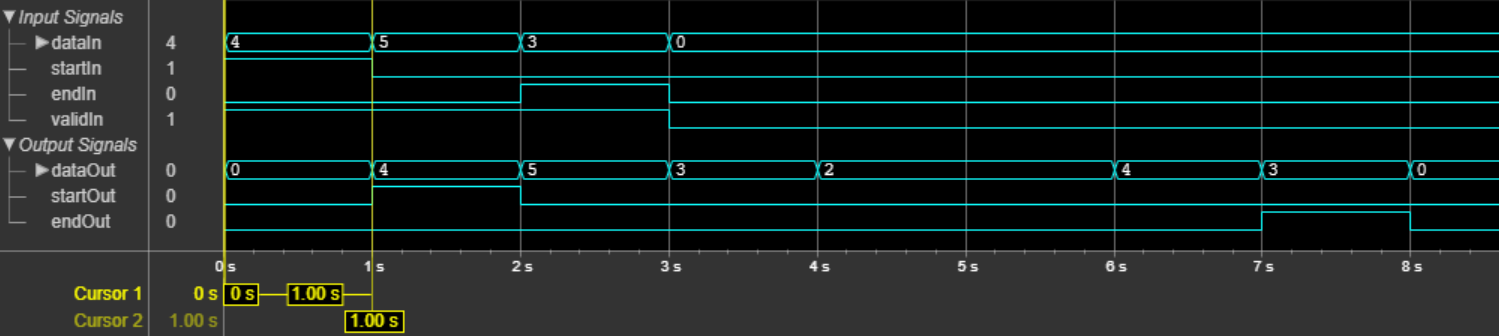Integer-Input RS Encoder HDL Optimized

Encode data using a Reed-Solomon encoder

• Library:
• Communications Toolbox HDL Support / Error Detection and Correction / Block

Communications Toolbox / Error Detection and Correction / Block

•Description

The Integer-Input RS Encoder HDL Optimized block encodes data using the RS encoder. The RS encoding follows the same standards as any other cyclic redundancy code. Use this block to model communications system forward error correction (FEC) codes. The block provides an architecture suitable for HDL code generation and hardware deployment.

Ports

Input

expand all

Input data, specified as a scalar representing one symbol. For binary point scaling, the input data type must be an integer or fixdt. The word length of each symbol must be equal to ceil(log2(Codeword length) + 1. The double data type is allowed for simulation, but not for HDL code generation.

Data Types: double | int8 | int16 | int32 | int64 | fixed point

Start of input frame indicator, specified as a Boolean scalar.

Data Types: Boolean

End of input frame indicator, specified as a Boolean scalar.

Data Types: Boolean

Valid input data indicator, specified as a Boolean scalar.

This is a control signal that indicates if the data on the dataIn port is valid.

Data Types: Boolean

Output

expand all

Output data, returned as a scalar. This output data width is the same as the input data width.

Data Types: double | int8 | int16 | int32 | int64 | fixed point

Start of output frame indicator, returned as a Boolean scalar.

Data Types: Boolean

End of output frame indicator, returned as a Boolean scalar.

Data Types: Boolean

Valid output data indicator, returned as a Boolean scalar.

This is a control signal that indicates if the data on the dataOut port is valid.

Data Types: Boolean

Parameters

expand all

Specify the codeword length.

The codeword length N must be an integer equal to 2M – 1, where M is an integer in the range from 3 to 16. For more information on representing data for RS codes, see Integer Format (Reed-Solomon Only).

Specify the length of the message.

For more information on representing data for RS codes, see Integer Format (Reed-Solomon Only).

Each input frame, that is, the number of valid data samples between startIn and endIn port values, must contain more than NK symbols and less than or equal to K symbols. A shortened code is inferred anytime the number of input data samples in a frame is less than K.

Specify the source of the primitive polynomial.

• Select Auto to specify the primitive polynomial based on the Codeword length parameter value. The degree of the primitive polynomial is calculated as M = ceil(log2(Codeword length). .

• Select Property to specify the primitive polynomial using the Primitive polynomial parameter.

Specify a binary row vector representing the primitive polynomial in descending order of powers.

For more information on how to specify a primitive polynomial, see Primitive Polynomials and Element Representations.

Dependencies

To enable this parameter, set the Source of primitive polynomial parameter to Property.

Select Property to enable the Puncture pattern vector parameter.

Specify a column vector of length NK. In a puncture vector, a value of 1 represents that the data symbol passes unaltered. A value of 0 represents that the data symbol is punctured, or removed, from the data stream.

Dependencies

To enable this parameter, set the Source of puncture pattern parameter to Property.

Specify the source of the starting power for roots of the primitive polynomial.

• Select Property to enable the B value parameter.

• Select Auto, to use the B value parameter default value of 1.

The starting exponent of the roots.

Dependencies

To enable this parameter, set the Source of B, the starting power for roots of the primitive polynomial parameter to Property.

Algorithms

This figure shows a sample output of the Integer-Input RS Encoder HDL Optimized block with a default configuration.